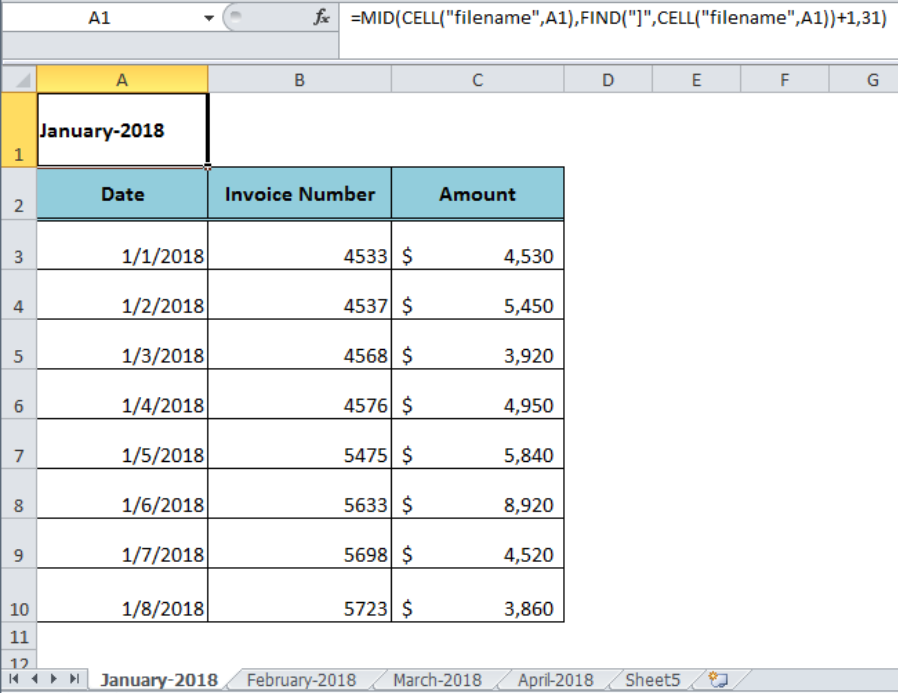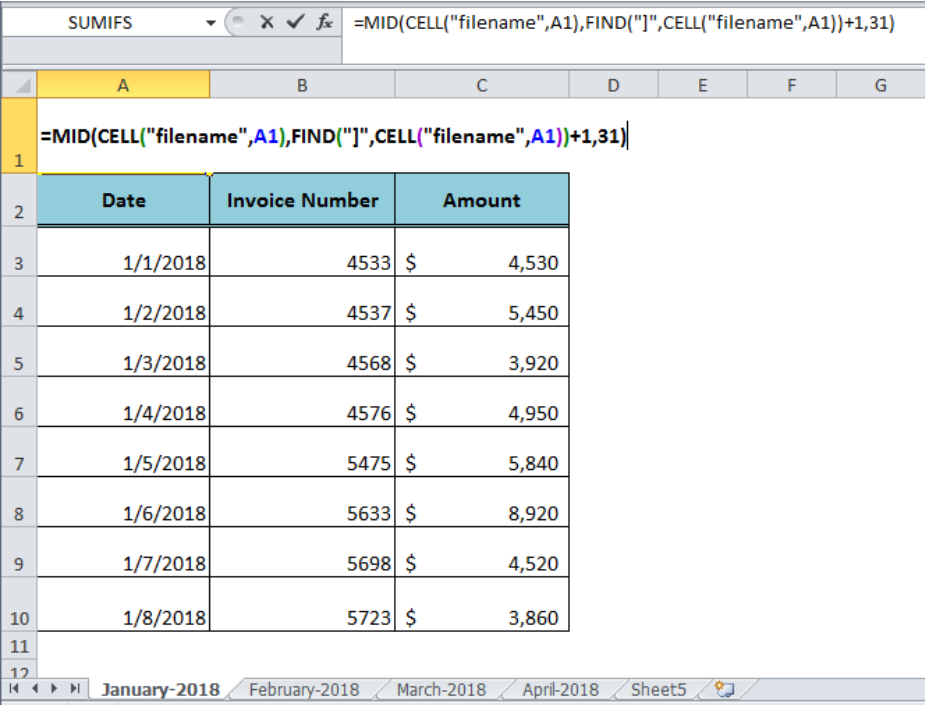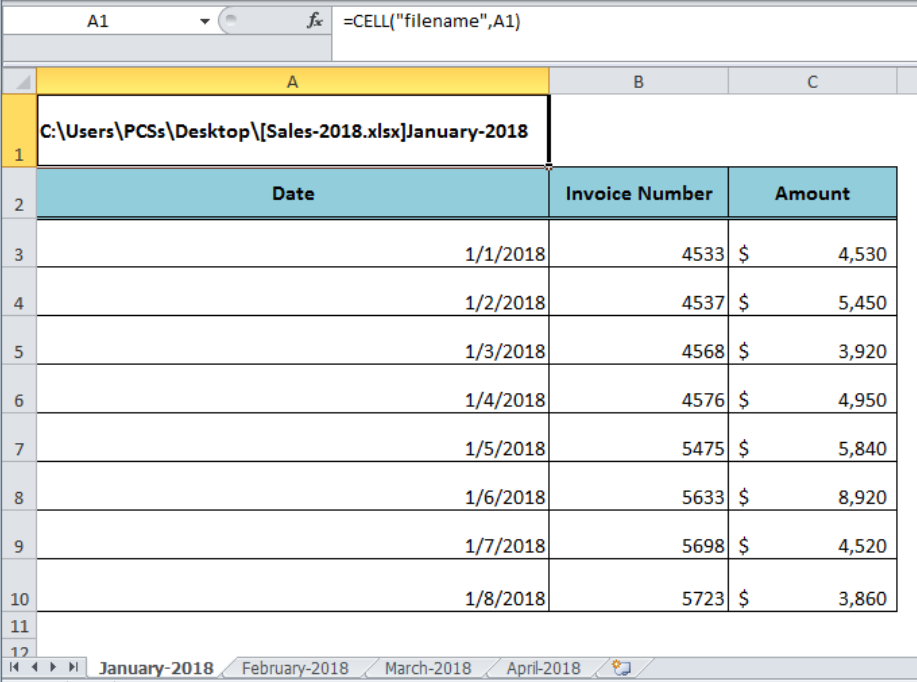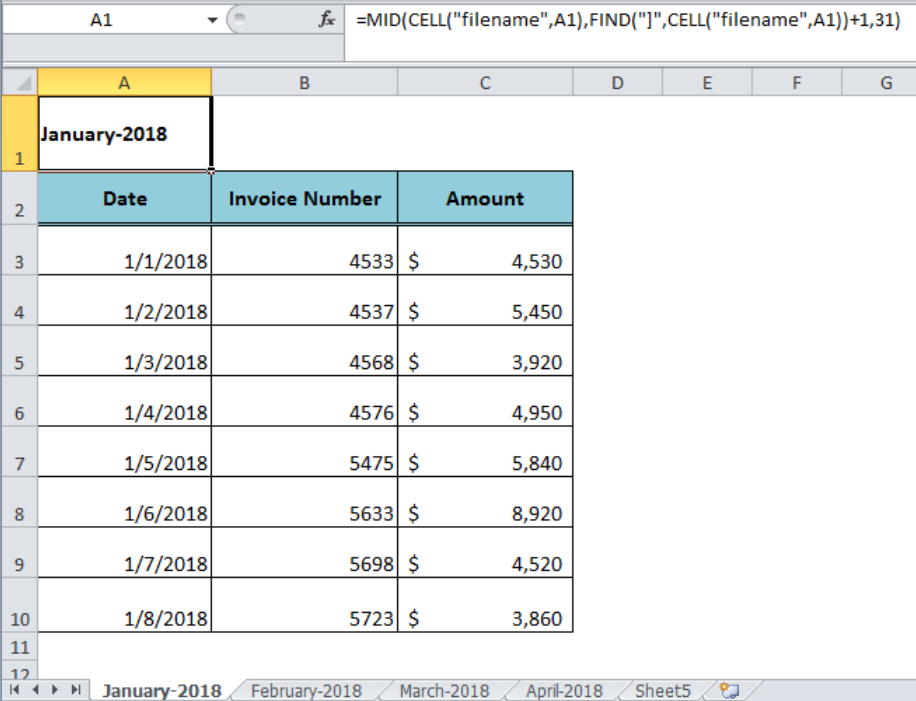Get instant live expert help with Excel or Google Sheets“My Excelchat expert helped me in less than 20 minutes, saving me what would have been 5 hours of work!”

#### Post your problem and you’ll get Expert help in seconds.

Your message must be at least 40 characters
Our professional Expert are available now. Your privacy is guaranteed.

# How to Get Sheet Name Only in Excel

When the Excel Workbook contains multiple worksheets and we want to use each worksheet name as the header in the worksheet without typing it again and again. This can be achieved by using a formula in a blank cell of each worksheet that returns the worksheet name only. The formula uses the Excel MID function along with CELL and FIND functions to get sheet name only.Figure 1. Getting Sheet Name Only

## Example

Suppose we have sales workbook that contains multiple worksheets. Each month’s sales data is kept in a separate sheet and it is named with month and year of sales, like January-2018, February-2018 etc. We want to get the name of the sheet in a blank cell, say cell A1, of every sheet to use as the header of that sheet. The following formula returns the sheet name only in cell A1, such as;

`=MID(CELL("filename",A1),FIND("]",CELL("filename",A1))+1,31)`Figure 2. The Formula to Get Sheet Name Only

## Working of the Formula

The CELL function with the info_type “filename” returns the full path, file name, and current sheet name when used in a cell of the saved workbook, such as;

`=CELL("filename",A1)`Figure 3. The CELL Function Result in Formula

The FIND function locates the position of the closing square bracket “]” character within the full path, file name, and current sheet name returned by the CELL function. We add 1 in the count to move the position by one character, such as;

`=FIND("]",CELL("filename",A1))+1`

And the MID function returns the portion of the text string starting from the position number returned by the FIND function as per the specified number of characters (31) as we cannot name a sheet longer than 31 characters.Figure 4. Final Preview of the Formula

## Instant Connection to an Expert through our Excelchat Service:

Most of the time, the problem you will need to solve will be more complex than a simple application of a formula or function. If you want to save hours of research and frustration, try our live Excelchat service! Our Excel Experts are available 24/7 to answer any Excel question you may have. We guarantee a connection within 30 seconds and a customized solution within 20 minutes.

Solution examplesI need Vlookup help across 2 workbooks into one other main workbook and can't figure out what i'm doing wrong!
Solved by G. E. in 19 minsI need a formula .... i need to decrease the premium an employee pays by 10% each year for ages 65-75. So when they are 65 it decreases 10%, age 66 it decreases 20% and so on. I tried =IF(OR(\$D2+1<65,\$D2+1=66,\$D2+2=77, ...... ),J2,J2-(J2*0.1),J2(J2*0.2) .... ) D2 being the current age of the employee, with each column being year 1, year 2, etc. However, excel will not allow me to do to IF D2+1=66, til age 75. It's too long. I know there has to be a better way! HELP!
Solved by F. F. in 60 minsI need to total a column of values that are in a custom format h:mm:ss; when you total it in a number format. If I change it to the same customer format I then need to deduct if from total hours of production. and does not look right I am wondering if my total hours worked which is a number value should be changed to look correct
Solved by K. W. in 60 minsCan you re edit this formula for me =IFERROR(IF(LEN(MID("RHS",1,FIND("RHS",B4,1)))=1,LEFT(B4,3),MID("RHS",1,FIND("RHS",B4,1))),MID("LHS",1,FIND("LHS",B4,1)))
Solved by S. L. in 40 minsI am not sure if my vlookup is working correctly. I have 30,000 ID to match but only 3,000 matched which makes me concern. I need to identify the duplicates and eliminate them.
Solved by V. E. in 60 mins